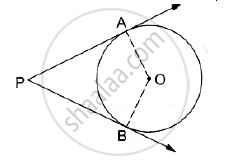# In the Given Figure, O is the Centre of the Circle. Pa and Pb Are Tangents. Show that Aobp is Cyclic Quadrilateral. - Mathematics

In the given figure, O is the centre of the circle. PA and PB are tangents. Show that AOBP  is cyclic quadrilateral.#### Solution

We know that the radius and tangent are perpendicular at their point of contact
∵ ∠OBP = ∠OAP = 90°
∠APB +∠AOB + ∠OBP + ∠OAP = 360° [Angle sum property of a quadrilateral]
⇒ ∠APB + ∠AOB + 90°  + 90°  = 360°
⇒∠APB + ∠AOB =180°
Since, the sum of the opposite angles of the quadrilateral is 180°
Hence, AOBP is a cyclic quadrilateral

Concept: Concept of Circle - Centre, Radius, Diameter, Arc, Sector, Chord, Segment, Semicircle, Circumference, Interior and Exterior, Concentric Circles
Is there an error in this question or solution?